×#### Thank you for registering.

One of our academic counsellors will contact you within 1 working day.

Click to Chat

1800-1023-196

+91-120-4616500

CART 0

• 0

MY CART (5)

Use Coupon: CART20 and get 20% off on all online Study Material

ITEM
DETAILS
MRP
DISCOUNT
FINAL PRICE
Total Price: Rs.

There are no items in this cart.
Continue Shopping• Complete JEE Main/Advanced Course and Test Series
• OFFERED PRICE: Rs. 15,900
• View Details

```Chapter 9: Ratio, Proportion and Unitary Method Exercise 9.2

Ratio, Proportion and Unitary Method – Exercise 9.2 – Q.1

(i) 3 : 4 (or) 9 : 16

Writing the given ratios as fractions, we haveNow L.C.M of 4 and is is 16

Making the denominaator of each fraction

equal to 16, we haveClearly 12 >9(ii) 15 : 16 or 24 : 24

Writing the given ratio as fractions, we have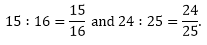L.C.M of 25 & 16 is = 400

Making the denominator of each fraction equal to 400, we haveclearly 384 > 375(iii) 4 :7 or 5 : 8Now, LCM of 7 and 8 is 56.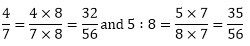Clearly 35 > 32(iv) 9 : 20 or 8 : 13.Now, LCM of 20 and 13 is 260Clearly 160 > 117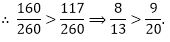(v) 1 : 2 or 13 : 27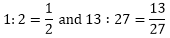Now, LCM of 2 and 27 is 54Ratio, Proportion and Unitary Method – Exercise 9.2 – Q.2

i.e. have.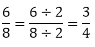∴  3 : 4 is an equivalent ratio of 6 : 15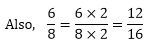So, 12 : 16 is an equivalent ratios of 6:8. Hence, 3 : 4 and 12 : 16 are equivalent ratios of 6 : 8.

Ratio, Proportion and Unitary Method – Exercise 9.2 – Q.3In order to find the first missing number, we consider the denominator 20 and 35

LCM of 20 and 5 is 20.

We have 20 ÷ 4 = 4.

So, we divide the Nr or of 12/20 by 4 to get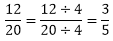Hence, first missing number is 3, consequently the second ratio is 3/5

To find the second missing number, we consider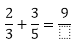We have 9 ÷ 3 = 3, so we multiply the nr ∆ or of 3/5 by 3 to getHence, the second missing number is 15.
```### Course Features

• 728 Video Lectures
• Revision Notes
• Previous Year Papers
• Mind Map
• Study Planner
• NCERT Solutions
• Discussion Forum
• Test paper with Video Solution